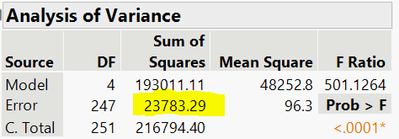Choose Language Hide Translation Bar
Highlighted

## How do I calculate partial r-squared on a multivariate regression model

Hello,

I have run a multivariate model on JMP, and am now trying to find the partial r-squared for each of my independent variables, but I am having some trouble figuring out how. Would anyone be able to help me answer these 2 questions:

1. Is "partial r-squared" the same as "Squared Partial Correlation Coefficients"?

2. How would I be able to calculate the partial r-squared?

Any help at all would be appreciated. Thank you

4 REPLIES 4
Highlighted

## Re: How do I calculate partial r-squared on a multivariate regression model

1. The partial correlation coefficient is a measure of the strength of a linear relationship between two variables after correlations with other variables is removed.
2. The partial R square is the coefficient of determination from a linear model attributed to a single predictor. See this Wikipedia entry for the description of the calculation. It is the ratio of the difference between the error sum of squares of the reduced model and the full model to the error sum of squares of the reduced model. The full model includes all terms. The reduced model includes all but the the term to be evaluated. So you fit the full model and recored the SSE (full). Remove the term in question and record the new SSE (reduced). Now compute (SSE (reduced) - SSE (full)) / SSE (reduced).
Learn it once, use it forever!
Highlighted

## Re: How do I calculate partial r-squared on a multivariate regression model

Thank you so much! This is really helpful.

Just to confirm, the SSE is the highlighted value we get from this table here, correct?And to make sure I understand your answer correctly, if, for example, I was running a multivariate model with 3 independent variables A, B, C to try and predict an outcome, then to calculate partial r2 for A:

1. I would run the full multivariate model with A, B, C and record the SSE.

2. Run the (reduced) multivariate model with variables B and C and record the SSE.

And then plug it into the formula?

Apologies for all the questions -- I'm quite new to JMP and regression modelling and want to make sure I fully understand

Highlighted

## Re: How do I calculate partial r-squared on a multivariate regression model

Yes and yes.

Learn it once, use it forever!
Highlighted

## Re: How do I calculate partial r-squared on a multivariate regression model

Great! Thank you for your help
Article Labels